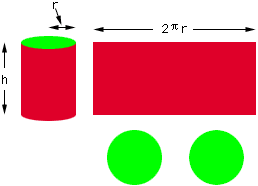Name: May Thin Zar Han Who is asking: Student Level: All Question: A can is to be made to hold 1 L of oil. Find the dimensions that will minimize the cost of the metal to manufacture the can. Hi, Suppose that the can has height h cm and the radius of the base is r cm. To determine the surface area (amount of metal) imagine that you cut the top and bottom from the can. Then slice upwards through the side of the can and roll it out to form a rectangle.The area of each of the ends is pi r2 and the area of the rectangle is 2 pi r h and hence the total area is A = 2 pi r2 + 2 pi r h. To minimize this expression you first have to express it as a function of one variable. You can do this by using the fact that the volume is 1 L, or 1000 cc. The volume of a can is pi r2 h and hence 1000 = pi r2 h. Use this expression to write A as a function of r and then apply the calculus you know to minimize A. Cheers, Harley Go to Math Central